Which Navier-Stokes equation do you use?

In the first half of 1999, a major turbulence programme was held at the Isaac Newton Institute in Cambridge. On those days when there were no lectures or seminars during the morning, a large group of us used to meet for coffee and discussions. In my view these discussions were easily the most enjoyable aspect of the programme. On one particular morning, as a prelude to making some point, I said that I was probably unusual in that I have taught the derivation of the Navier-Stokes equation (NSE) as continuum mechanics to engineering students and by statistical mechanics to physicists and mathematicians. The general reaction was that that I was not merely unusual, but surely unique! I gathered, from comments made, that everyone present saw the NSE as part of continuum mechanics.

Of course the two forms of NSE are apparently identical, otherwise one could not refer to both as the Navier-Stokes equation. Nevertheless, when one comes to consider the infinite Reynolds number limit, it is necessary to become rather more particular. We can start doing this by stating the two forms, as follows.

First, the continuum-mechanical NSE is exact for a continuous fluid which shows Newtonian behaviour under all circumstances of interest.

Secondly, the statistical-mechanical NSE is the first approximation to the exact statistical mechanical equations of motion. So in principal it should be followed by a statement to the effect that there are higher-order terms.

Now strictly, if we want to consider cases where the continuum approximation breaks down, we should be using the second of these forms. Batchelor argued that in the limit of zero viscosity (at constant dissipation rate) the dissipation would be concentrated at infinity in wavenumber space. Edwards  went further and represented this dissipation by a delta-function at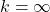and matched it with a delta-function input of energy at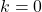. In this way he could obtain an infinitely long inertial range and assume that the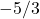spectrum applied everywhere, as a test of his closure approximation.

The Edwards procedure is valid, because he was applying it to a closure of the (in effect) continuum-mechanical NSE, as indeed is everyone else who discusses behaviour at large Reynolds numbers; or, for that matter, statistical closures. But the question of the validity of this model arises when people consider the breakdown of the NSE. This actually requires some consideration of the basic physics, which in this case means statistical mechanics; and, essentially this boils down to the following: The general requirement for the continuum limit to be valid is that the smallest length-scale of the fluid motion should be much larger than the mean free path of the fluid’s molecules.

The only example of this being looked at quantitatively, that I know of, may be found in Section 1.3 of the book by Leslie . He considered flow in a pipe at a Reynolds number of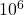, with a pipe diameter of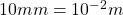, which he described as an extreme case. In Section 2.8 of his book, he calculates the minimum eddy size to be greater than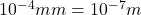. He notes that for a liquid the mean free path is of the order of the atomic dimensions and thus about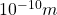and hence the use of a continuum form is very well justified. He further comments: ‘It [the continuum limit] is also satisfied, although not by such a comfortable margin, by any gas dense enough to produce a Reynolds number ofin a passage only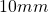in diameter.’

I think that it would be a good idea if those who discuss cases where a theory based on the Navier-Stoke equation is supposed to break down actually put in some numbers to indicate where their revised theory would be applicable and the NSE wouldn’t. Or perhaps, it might be salutary to consider in detail the variation of significant quantities with increasing Reynolds number and identify the smooth development of asymptotic behaviour. I will return to this point in future posts.

Anyone who would like an introductory discussion of the derivation of macroscopic balance equations from statistical mechanics should consult Section 7.6 of my book Study notes for statistical physics, which may be downloaded free of charge from Bookboon.com.

 S. F. Edwards. Turbulence in hydrodynamics and plasma physics. In Proc. Int. Conf. on Plasma Physics, Trieste, page 595. IAEA, 1965.
 D. C. Leslie. Developments in the theory of turbulence. Clarendon Press, Oxford, 1973.